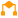# If

 Block Group: Logic Icon:The If block returns one value if a condition that you specify evaluates to TRUE and another value if the condition evaluates to FALSE.

For information on using dataflow blocks, see Dataflow.

## Input/Output Properties

The following properties of the If block can take input and give output.

• op (enum)
• input n
• then
• else

op specifies the arithmetic, comparison, or text operator to apply to the input values. It is used in conjunction with input n to form an expression that can be evaluated to TRUE or FALSE. The value of the op property can be one of the following:

• = (equal to) – The expression evaluates to TRUE if all input values are equal.
• > (greater than) – The expression evaluates to TRUE if each input value is greater than the value that immediately follows it.
• < (less than) – The expression evaluates to TRUE if each input value is less than the value that immediately follows it.
• >= (greater than or equal to) – The expression evaluates to TRUE if each input value is greater than or equal to the value that immediately follows it.
• < = (less than or equal to) – The expression evaluates to TRUE if each input value is less than or equal to the value that immediately follows it.
• != (not equal to) – The expression evaluates to TRUE if all input values are different. No two can be equal.
• and – The expression evaluates to TRUE if all input values evaluate to TRUE. See also: And block.
• or – The expression evaluates to TRUE if one or more input values evaluate to TRUE. See also: Or block.

input n specifies two or more values to be evaluated using the operator specified.

then specifies the value to return if the expression evaluates to TRUE.

else specifies the value to return if the expression evaluates to FALSE.

## Output Property

The following property of the If block can give output but cannot take input.

• output (number, string or boolean)

output returns either the then value or the else value. The then value is returned if the expression evaluates to TRUE, and the else value is returned otherwise.

## Examples

The following image demonstrates two examples of the If block. In this image, the leftmost If block returns “Within Budget” if the bound value is less than 100 and returns “Over Budget” otherwise. The rightmost If block returns twice the value of input 0 if input 0 is less than 100 and returns 100 otherwise.